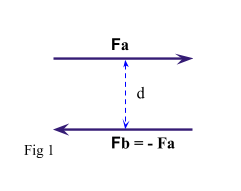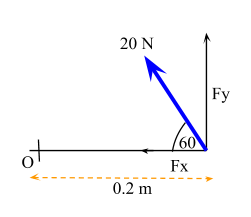# Moments

Contents

## Summary

• A moment is the turning effect a force has around a point.
• In order for a moment to develop, the force must act upon the body in such a manner that the body would begin to twist.
• Moment = Force X Perpendicular Distance
• For a body or particle in equilibrium the total moments either way must be equal.
• Remember that the weight of anything always acts at its centre of mass. E.g for a uniform beam, the centre of mass is always at the exact centre of the beam.

#### What is a Moment?

A moment is the turning effect a force has around a point. It is basically the tendency to cause a body to rotate about a specific point or axis. This is not the same as when force is applied to a body it moves, or translates, in the direction of the force. In order for a moment to develop, the force must act upon the body in such a manner that the body would begin to twist.

The moment of force acting on an object, often called torque, is the product of the force and the distance to the object (i.e., the reference point).

Moment is defined using the following formula:

Moment = Force X Perpendicular Distance

You must note that in equilibrium moments total to zero. In other words for a body or particle in equilibrium the total moments either way must be equal.

Total Clockwise Moment = Total Anticlockwise Moment

Another important thing to study in this topic is couples. Couple is a pair of forces, equal in magnitude, oppositely directed, and displaced by perpendicular distance. Since the forces are equal and oppositely directed, the resultant force is zero. But the displacement of the force couple (d) in Fig 1 will create a couple moment.#### Example #1

Q. A force of 20 N is applied to a spanner, attached to a bolt at a point O. The force is applied at an angle of 60° to the spanner head as shown. Find the turning effect of the force upon the bolt.Solution:

The 20 N force here can be resolved into two components:${ F }_{ x }$  and${ F }_{ y }$

As we can see that${ F }_{ x }$ goes through O, so its moment will be zero.

Therefore, we only work out${ F }_{ y }$.${ F }_{ y }\quad =\quad 20sin(60)$

We use the formula discussed above to work out the moment.

Moment = Force X Perpendicular Distance

Moment = 20 sin (60) X 0.2

Moment = 3.46 Nm          Ans

Before we move on to our next example remember that the weight of anything always acts at its centre.

E.g for a uniform beam, the centre of mass is always at the exact centre of the beam. The example below shows how to take this into consideration when solving questions on moments.

#### Example #2

Q. A 6m long uniform beam AB of weight 40 N is supported at A by a vertical reaction R. AB is held horizontal by a vertical wire attached 1m from the other end. A particle of weight of 30 N is placed 2m from the support R. Find the tension T in the wire and the force R.Solution:

We start off by taking moments around the point of action of one of the unknown forces. Hence we first take moments about A.

We know that:

Total Clockwise Moment = Total Anticlockwise Moment

(30 X 2) + (40 X 3) = T X 5
T = 36 N

Now resolving vertically:

T + R = 30 + 40
As T = 36 N
R = 34 N      Ans

##### Reference
1. CGP As Level Mathematics complete revision and practice Edexcel
2. http://web.mit.edu/4.441/1_lectures/1_lecture5/1_lecture5.html
3. https://en.wikipedia.org/wiki/Moment_(physics)
4. http://www.secs.oakland.edu/~latcha/EGR280/05-Moment.pdf